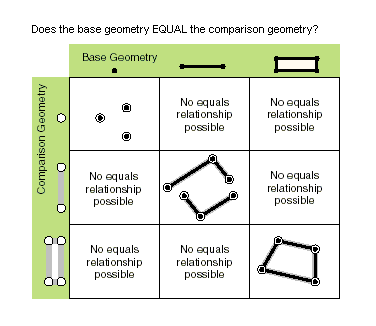This document is archived and information here might be outdated.  Recommended version.

IRelationalOperator.Equals Method (ArcObjects .NET 10.8 SDK)
 ArcObjects Help for .NET developers > ArcObjects Help for .NET developers > ArcObjects namespaces > Geometry > ESRI.ArcGIS.Geometry > Interfaces > IR > IRelationalOperator Interface > IRelationalOperator.Equals Method
 ArcGIS Developer Help

# IRelationalOperator.Equals Method

Indicates if the two geometries are of the same type and define the same set of points in the plane.

```[Visual Basic .NET]
Public Function Equals ( _
ByVal other As IGeometry _
) As Boolean
```
```[C#]
public bool Equals (
IGeometry other
);
```
```[C++]
HRESULT Equals(
IGeometry* other
);
```
```[C++]
Parameters
other
other is a parameter of type IGeometry*
```

#### Product Availability

Available with ArcGIS Engine, ArcGIS Desktop, and ArcGIS Server.

#### Description

For polylines and polygons, two geometries are equal when their symmetric difference is the empty set.  Note, orientation and M/Z attributes are not considered here.  Equals is not a Clementini operator. For multipoints, IRelationalOperator::Equals delegates to IClone::IsEqual.

#### Remarks

Only geometries that support the IRelationalOperator interface can be used as input geometries. GeometryBags are not supported. IRelationalOperator::Equals delegates to ITopologicalOperatorSymmetricDifference and returns true when that method returns an empty geometry; M's and Z's are ignored. Please use IClone::IsEqual if M's and Z's need to be compared.

IClone::IsEqual simply iterates over each segment in each geometry, in index order, and compares their types and coordinates (but using the spatial reference's cluster tolerances to determine equality of coordinates).

Only "true" relationships are showed in this picture.[C#]

The RCW for the IRelationalOperator inherits from System.Object, as do all reference types in .NET. The System.Object type has a method called Equals, which results in the IRelationalOperator RCW having two overloaded versions of the Equals method. Ensure that you are not using the Equals method inherited from the System.Object class by mistake, by making sure you pass in an IGeometry reference to the Equals method. For example, the following extract of code from a C# console application demonstrates calling Equals correctly.

``````ESRI.ArcGIS.Geometry.IPoint pt1 = new ESRI.ArcGIS.Geometry.PointClass();
pt1.PutCoords(5, 5);

ESRI.ArcGIS.Geometry.IPoint pt2 = new ESRI.ArcGIS.Geometry.PointClass();
pt2.PutCoords(5, 5);

ESRI.ArcGIS.Geometry.IRelationalOperator rel1 = pt1 as ESRI.ArcGIS.Geometry.IRelationalOperator;
ESRI.ArcGIS.Geometry.IGeometry geom2 = pt2 as ESRI.ArcGIS.Geometry.IGeometry;

bool relEqual = rel1.Equals(pt2); //calling IRelationalOperator::Equals
Console.WriteLine("Equal: " + relEqual.ToString());
bool netEqual =  rel1.Equals(pt2 as object);  //calling Object::Equals
Console.WriteLine("Equal: " + netEqual.ToString());
```
```